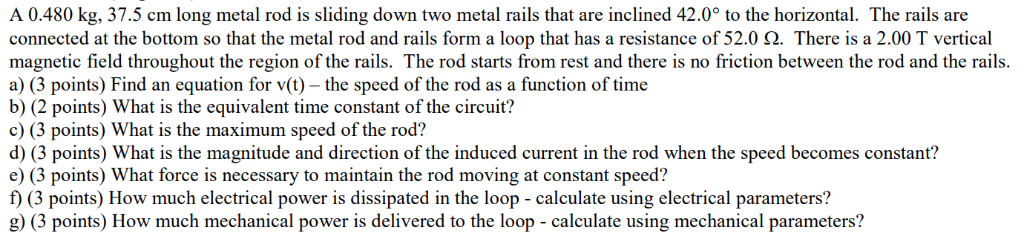# A 0.480 kg, 37.5 cm long metal rod is sliding down two metal rails that are...

###### Question:A 0.480 kg, 37.5 cm long metal rod is sliding down two metal rails that are inclined 42.0° to the horizontal. The rails are connected at the bottom so that the metal rod and rails form a loop that has a resistance of 52.0 Ω. There is a 2.00 T vertical magnetic field throughout the region of the rails. The rod starts from rest and there is no friction between the rod and the rails a) (3 points) Find an equation for v(t) - the speed of the rod as a function of time b) (2 points) What is the equivalent time constant of the circuit? c) (3 points) What is the maximum speed of the rod? d) (3 points) What is the magnitude and direction of the induced current in the rod when the speed becomes constant? e) (3 points) What force is necessary to maintain the rod moving at constant speed? f) (3 points) How much electrical power is dissipated in the loop - calculate using electrical parameters? g) (3 points) How much mechanical power is delivered to the loop - calculate using mechanical parameters?